Jim Simons

•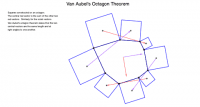Van Aubel's Octagon Theorem

Activity

Jim Simons

•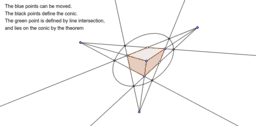Chern's Box Theorem

Activity

Jim Simons

•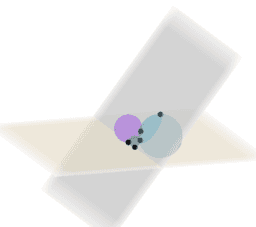Chern's Sphere Theorem

Activity

Jim Simons

•Parallelograms in perspective

Activity

Jim Simons

•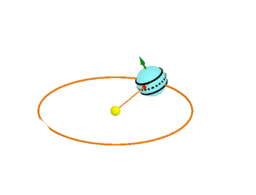Earth Sun system

Activity

Jim Simons

•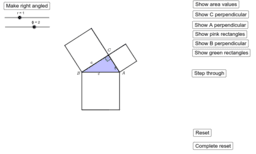Demonstrate generalisation of Pythagoras

Activity

Jim Simons

•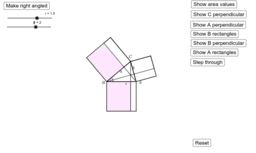First proof of Pythagoras generalisation

Activity

Jim Simons

•Euclid's proof of Puythagoras generalised

Activity

Jim Simons

•Generalising Pythagoras

Book

Jim Simons

•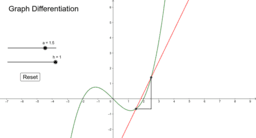The Chain Rule - How to really Understand it

Book

Jim Simons

•Earth and Sun

Activity

Jim Simons

•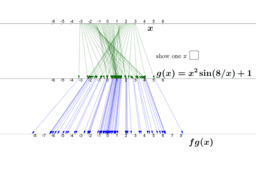Chain Rule for x^2 sin(8/x)

Activity

Jim Simons

•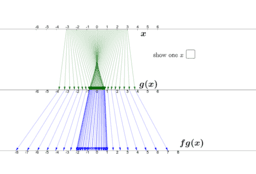Chain rule using the mapping view

Activity

Jim Simons

•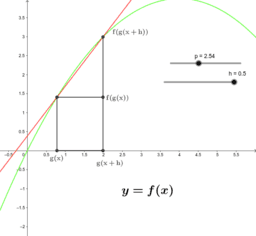Chain rule using the graph view

Activity

Jim Simons

•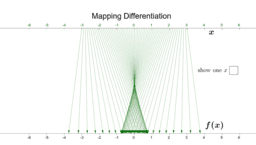Differentiation Using the Mapping View

Activity

Jim Simons

•Differentiation Using the Graph View

Activity

Jim Simons

•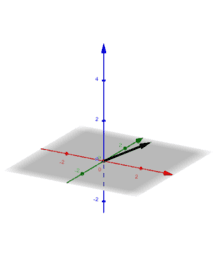1-Dimensional Vectors

Book

Jim Simons

•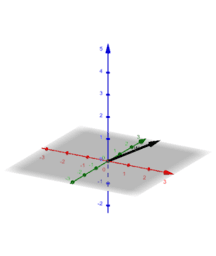negating vectors

Activity

Jim Simons

•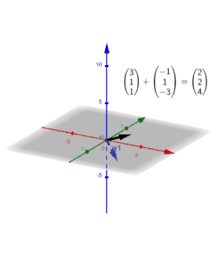subtracting vectors

Activity

Jim Simons

•moving vectors

Activity

Jim Simons

•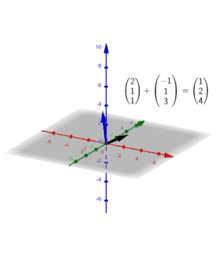Activity

Jim Simons

•Graph Transformations

Book

Jim Simons

•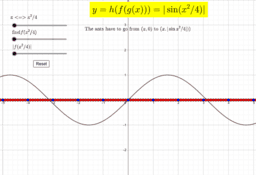|sin(x^2/4)| from sin(x)

Activity

Jim Simons

•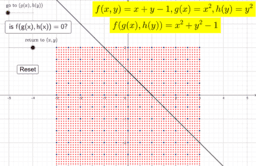Activity

Jim Simons